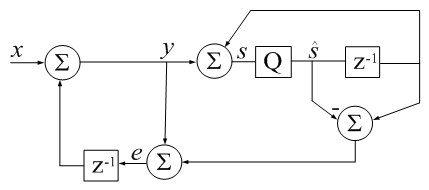# Accumulator Error Feedback

(Difference between revisions)
 Revision as of 19:20, 25 September 2017 (edit)← Previous diff Revision as of 19:23, 25 September 2017 (edit) (undo)Next diff → Line 18: Line 18: % % % clear all % clear all - % % v=sort(randn(13e6,1),'descend'); %better when sorted + % % v = sort(randn(13e6,1),'descend'); %better when sorted - % v=randn(13e6,1); + % v = randn(13e6,1); % rsumv = abs(sum(v) - sum(v(end:-1:1))); % rsumv = abs(sum(v) - sum(v(end:-1:1))); % disp(['rsumv = ' num2str(rsumv,'%18.16f')]); % disp(['rsumv = ' num2str(rsumv,'%18.16f')]);

## Revision as of 19:23, 25 September 2017CSUM() in Digital Signal Processing terms: z-1 is a unit delay, Q is a floating-point quantizer to 64 bits, qi represents error due to quantization (additive by definition).
-Jon Dattorro
```function s_hat=csum(x)
% CSUM Sum of elements using a compensated summation algorithm.
%
% For large vectors, the native sum command in Matlab does
% not appear to use a compensated summation algorithm which
% can cause significant roundoff errors.
%
% This code implements a variant of Kahan's compensated
% summation algorithm which often takes about twice as long,
% but produces more accurate sums when the number of
% elements is large. -David Gleich
%
%
%  clear all
% % v = sort(randn(13e6,1),'descend');             %better when sorted
%  v = randn(13e6,1);
%  rsumv = abs(sum(v) - sum(v(end:-1:1)));
%  disp(['rsumv = ' num2str(rsumv,'%18.16f')]);
%  csumv = abs(csum(v) - csum(v(end:-1:1)));
%  disp(['csumv = ' num2str(csumv,'%18.16f')]);
% % vsumv = sum(vpa(v)) - sum(vpa(v(end:-1:1))); %vpa toolbox 32GB RAM
% % disp(['vsumv = ' char(vsumv)])

s_hat=0; e=0;
for i=1:numel(x)
s_hat_old = s_hat;
y = x(i) + e;
s_hat = s_hat_old + y;
e = (s_hat_old - s_hat) + y;  %calculate difference first (Higham)
end
```